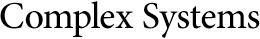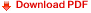## A Measure for the Complexity of Elementary Cellular AutomataThorsten Ewert
Lübeck, Germany
tewert@web.de

#### Abstract

A new measure for the complexity of elementary cellular automata (ECAs) is presented. This measure is based on the minimization of Boolean functions with three variables that represent the elementary cellular automaton (ECA) rules. The minimized Boolean functions reduce the number of input bits of the truth table, which is equivalent to the rule table of an ECA. This results in a fractalized number of Boolean variables that are equal to the state variables of a dynamic system. Furthermore, the dynamic nature of complexity in ECAs is considered. Therefore, a new method of defining and deriving the complexity of all 256 ECA rules given in bits is proposed. The results then can be described, classified and grouped. As for other continuous or discrete dynamic systems, the complexity grows with the number and the usage of the state variables. In ECAs, the numbers of the effective state variables range from 0 to 3, resulting in four classes of behavior.

Keywords: complexity; measure; elementary cellular automaton